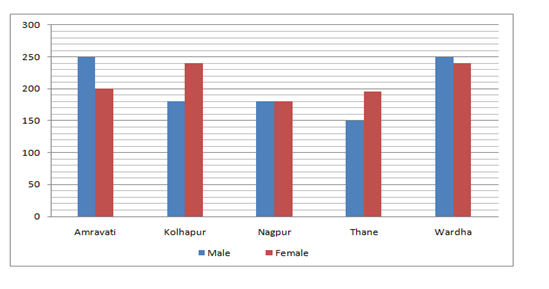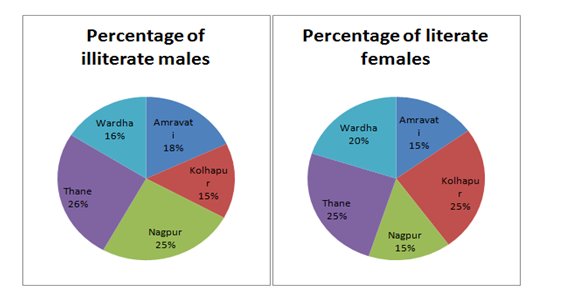# “20-20” Quantitative Aptitude | Crack SBI Clerk 2018 Day-12

Dear Friends, SBI Clerk 2018 Notification has been released we hope you all have started your preparation. Here we have started New Series of Practice Materials specially for SBI Clerk 2018. Aspirants those who are preparing for the exams can use this “20-20” Quantitative Aptitude Questions.

[WpProQuiz 1185]

Click “Start Quiz” to attend these Questions and view Explanation

### Click Here for SBI Clerk Prelims and Mains 2018 – Full Length Mock Test

Directions (Q. 1-5): What will come in place of question mark (?) in the following questions?

1. 0.2 × 1.1 + 0.6 × 0.009 = ? – 313.06
1. 349.3684
2. 333.4754
3. 320.1574
4. 313.2854
5. 303.2754
1. 7072 ÷ (16% of 884) = 30 × 1 3/12 of (? ÷ 39)
1. 60
2. 62
3. 65
4. 55
5. 67
1. (13 + 2 √5)^2 = ? √5 +189
1. 66
2. 36
3. 52
4. 44
5. 58
1. (562.5 × 6)^6 ÷ (135 ÷ 9)^10 ÷ (37.5 × 6)^7 = (3.75 × 4)^(? – 6)
1. 5
2. 3
3. 6
4. 1
5. 0
1. 1478.4 ÷ 56 + 66.8 × 57 = (? × 3) + (34 × 34.5)
1. 772
2. 887
3. 863
4. 925
5. 825

Directions (Q. 6-10): In each of these questions a number series is given. In each series only one number is wrong. Find out the wrong number.

6).   50,    51,    47,    56,    42,    65,    29

1. 51
2. 47
3. 56
4. 42
5. 65

7).   3,   9,   23,   99,   479,   2881,   20159

1. 9
2. 23
3. 99
4. 479
5. 2881

8).    7,    4,    6,    9,    20,    52.5,    160.5

1. 6
2. 4
3. 20
4. 9
5. 52.5

9).   1,    3,    6,   11,    20,    39,    70

1. 3
2. 39
3. 11
4. 20
5. 6

10).    2,    13,    27,    113,    561,    3369,    23581

1. 27
2. 13
3. 113
4. 561
5. 3369
1. Two pipes M and N can fill a cistern in 24 min and 32 min respectively. If both pipes are opened together, then after how many minutes N should be closed so that the tank is full in 18 minutes?
1. 6
2. 10
3. 8
4. 21
5. None of these
1. A shop keeper sold a TV set for Rs. 17940 with a discount of 8% and gained 19.6%. If no discount is allowed, then what will be his gain percentage?
1. 25
2. 30
3. 35
4. 26.4
5. None of these
1. Since price of the Banana goes down by 20% a man can now buy 2 bananas more in 40 Rs. What was the original price of one banana?
1. 25
2. 24
3. 23.5
4. 26
5. None of these
1. A man driving his bike at 24kmph reaches his office 5 minutes late. Had he driven 25% faster on an average he would have reached 4 minutes earlier than the scheduled time. How far is his office?
1. 24km
2. 72km
3. 18km
4. 56km
5. None of these
1. 40% of the employees in a factory are workers. All the remaining employees are executives. The annual income of each worker is 390rs. The annual income of each executive is Rs. 420. What is the average annual income of all the employees in the factory together?
1. 390
2. 405
3. 408
4. 415
5. None of these

Directions (Q. 16-20): Following graph shows the number of males and females in five different districts in Maharashtra.1. What is the total number of illiterate females in Nagpur District?
1. 150
2. 163
3. 143
4. 153
5. 133
1. The illiterate females in Amravati District is what per cent more than that in Thane District (take number of girls in thane=196)?
1. 11.8%
2. 15.6%
3. 12.8%
4. 17.2%
5. 16.9%
1. What is the total number of literate males?
1. 714
2. 906
3. 844
4. 794
5. 814
1. The literate females in Kolhapur District is what per cent more than the illiterate males in Nagpur District?
1. 17.33%
2. 45.66%
3. 50.33%
4. 66.22%
5. 33.33%
1. What is the total number of illiterate females (take number of girls in thane=196)?
1. 802
2. 838
3. 842
4. 876
5. 854

Directions (Q. 1-5):

0.22 + 0.0054 = ? – 313.06

0.2254 + 313.06 = ?

? = 313.2854

7072 ÷ (884 × 16/100)= 30 × 13/12 × ?/39

7072 ÷ 141.44 = 5 × ?/6

50 × 6 = 5 × ?

? = 50 × 6/5

= 60

(13+ 2 √5)^2 = ? × √5+ 189

169 + 20 + 2 × 13 × 2 √5 = ? × √5 + 189

189 + 52 × √5 = ? × √5 + 189

? = 52

(3375)^6 ÷ (15)^10 ÷ (225)^7 = (15)^(? – 6)

((15)^3)^6 ÷ (15)^10 ÷ (15^2)^7 = (15)^(? – 6)

15^(18–10–14) = (15)^(?–6)

– 6 = ? – 6

? = 0

1478.4/56 + 66.8 × 57 = ? × 3 + 34 × 34.5

26.4 + 3807.6 = ? × 3 + 1173

3834 – 1173 = ? × 3

? × 3 = 2661

? = 887

Directions (Q. 6-10):

The series is 50 + 1^2 = 51, 51 – 2^2 = 47, 47 + 3^2 = 56, 56 – 4^2 = 40, 40 + 5^2 = 65, 65 – 6^2 = 29.

Hence, there should be 40 in place of 42.

The series is 3 × 2 + 3 = 9, 9 × 3 – 4 = 23, 23 × 4 + 5 = 97, 97 × 5 – 6 = 479, 479 × 6 + 7 = 2881, 2881 × 7 – 8 = 20159

Hence, there should be 97 in place of 99.

The series is x0.5 + 0.5, x1 + 1, x 1.5 + 1.5, x 2 + 2, x 2.5 + 2.5, x 3 + 3…

Hence, there should be 5 in place of 6.

The series is 1 × 2 + 1 = 3, 3 × 2 + 0 = 6, 6 × 2 – 1 = 11, 11 × 2 – 2 = 20, 20 × 2 – 3 = 37, 37 × 2 – 4 = 70.

Hence, there should be 37 in place of 39.

The series is 2 × 2 + 7 = 11, 11 × 3 – 6 = 27, 27 × 4 + 5, = 113, 113 × 5 – 4 = 561, 561 × 6 + 3 = 3369, 3369 × 7 – 2 = 23581.

Hence, there should be 11 in place of 13.

Consider M & N pipes opened together for x min

M——24min         total work

N——-32min             96 units

Efficiency of M is 4

Efficiency of N is 3

7x+4(18-x) = 96

X = 8 minutes

SP=17940

d%=8%

therefore Marked Price(MP)=17940 x 100/92=19500

P% = 19.6%

CP=17940 x 100/119.6 = 15000

If no discount allowed on MP then SP=19500

P% = 4500 x 100/15000 = 30%

2 Bananas 40 Rs

1 banana 20 Rs

(80/100)X = 20

X= 25

Original price for 1 banana = Rs. 25

Let x km be the distance

Time1 = x/24 hrs

While travelling at 25% faster speed

24 + 25% 24 = 30 kmph

Time2= x/30 hrs

Total time difference 9 minutes

x/24 – x/30= 9

x = 18km

Let total No. of employees be 100

(40 x 390 + 60 x 420)/100 =  408

Directions (Q. 16-20):

The number of illiterate females of Nagpur District

=180 × 85 / 100 = 153

Illiterate females in Amravati District = 200 × 85 / 100 = 170

Illiterate females in Thane District = 196 × 75 / 100 = 147

Required % = (170 –147) / 147 × 100

= 15.6%

Total number of literate males

= {(250 × 82 / 100) + (180 × 85 / 100) + (180 × 75 / 100) + (150 × 74 / 100) + (250 × 84 / 100)}

= 205 + 153 + 135 + 111 + 210

= 814

The number of literate females of Kolhapur District = 240 × 25 / 100 = 60

The number of illiterate males of Nagpur District = 180 × 25 / 100 = 45

Required % = (60 – 45) / 45 × 100

= 15 / 45 × 100

= 33.33%

Total number of illiterate females of all districts together

= {(200 × 85 / 100) + (240 × 75 / 100) + (180 × 85 / 100) + (196 × 75 / 100) + (240 × 80 / 100)}

= 170 + 180 + 153 + 147 + 192

= 842